# Mathematics Paper 2 Questions and Answers - Kassu Joint Mock Examination 2021

INSTRUCTIONS

• Write your name, class, admission number, school, date and signature in spaces provided above.
• The paper contains two sections A and B.
• Answer all questions in section A and any five questions from section B in the spaces provided below each question.
• Show all the steps in your calculations giving your answers at each stage in the spaces below each question.
• Non-programmable silent electronic calculator and mathematical tables may be used except where stated otherwise.

SECTION A (50 MARKS)
(Answer all questions in this section in the spaces provided)

1. Use logarithm table to evaluate. (4mks)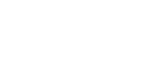2. Three sisters, Ann, Beatrice and Caroline together invested Ksh. 48,000 as capital and started a small business. If the share of profit is Ksh. 2,300, Ksh. 1,700 and Ksh. 800 respectively, shared proportionally. Find the capital invested by each of them. (3mks)
3. Make t the subject of formula in x=(p+t)1/3 (3mks)
t
4. Without using a calculator or mathematical tables, express      √3      in surd form and simplify. (3mks)
1 - cos30º
5. Expand and simplify (3x-y)4 hence use the first three terms of the expansion to approximate the value of (6-0.2)4. (4mks)
6. Find x without using tables if 3 + log2⁡3 + log2⁡x = log2⁡5 +2 (3mks)
7. Find the value of m for which the matrix transforms an object into a straight line. (3mks)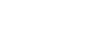8. In the figure below PT is a tangent to the circle at T, PQ = 9cm, SA = 6cm, AT = 8cm and AR = 3cm. Calculate the length of;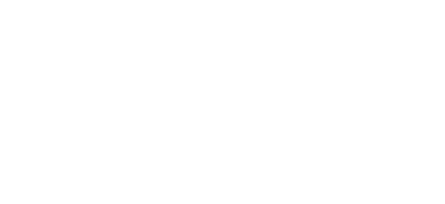1. AQ (2mks)
2. PT (1mk)
9. A right angled triangle has a base of 15.3 cm and height 7.2 cm, each measured to the nearest 3 mm. Determine the percentage error in finding the area of the triangle, giving your answer to 2 decimal places. (3mks)
10. Given that sin x=0.8, without using a mathematical table and calculator find tan(90-x) (3mks)
11. The point B(3,2) maps onto B1(7,1) under a translation T1. Find T1 (2mks)
12. Using a ruler and a pair of compasses only, construct triangle ABC in which BC=6cm, AB= 8.8cm and angle ABC= 22.5º. (3mks)
13. Two grades of tea A and B, costing sh 100 and 150 per kg respectively are mixed in the ratio 3:5 by mass. The mixture is then sold at sh 160 per kg. Find the percentage profit on the cost price. (3mks)
14. The first, the third and the ninth term of an increasing AP, makes, the first three terms of a G.P. If the first term of the AP is 3, find the difference of the AP and common ratio of GP. (4mks)
15. The matrix M=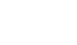maps a triangular object of area 7 square units onto one with area of 35 square units. Find the value of x. (4mks)
16. The equation of a circle is given by x2+4x+y2-2y-4=0. Determine the centre and radius of the circle (3mks)

SECTION B (50 MARKS)
(Answer any five questions in this section)

1. A bag contains 3 black balls and 6 white balls. If two balls are drawn from the bag one at a time, find the:
1. Probability of drawing two white balls:
1. With replacement (2mks)
2. Without replacement (2mks)
2. Probability of drawing a black ball and white ball:
1. With replacement (3mks)
2. Without replacement. (3mks)
2. In the triangle below P and Q are points on OA and OB respectively such that OP:PA = 3 : 2 and OQ : QB = 1 : 2. AQ and PQ intersect at T. Given that OA = a and OB = b.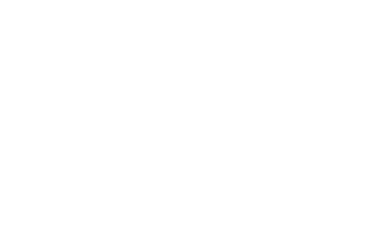1. Express AQ and PQ in terms of a and b. (2mks)
2. Taking BT=kBP and AT=hAQ where h and k are real numbers.
1. Find two expressions for OT in terms of a and b. (2mks)
2. Use the expression in b(i) above to find the values of h and k. (4mks)
3. Give the ratio BT:TP. (2mks)
3. Complete the table below for the functions y=3cosx-2 for 0º≤x≤360º (2mks)
 x 0 30 60 90 120 150 180 210 240 270 300 330 360 y=3cosx-2
1. Plot the graph of y=3cosx-2 in the graph provided below. (3mks)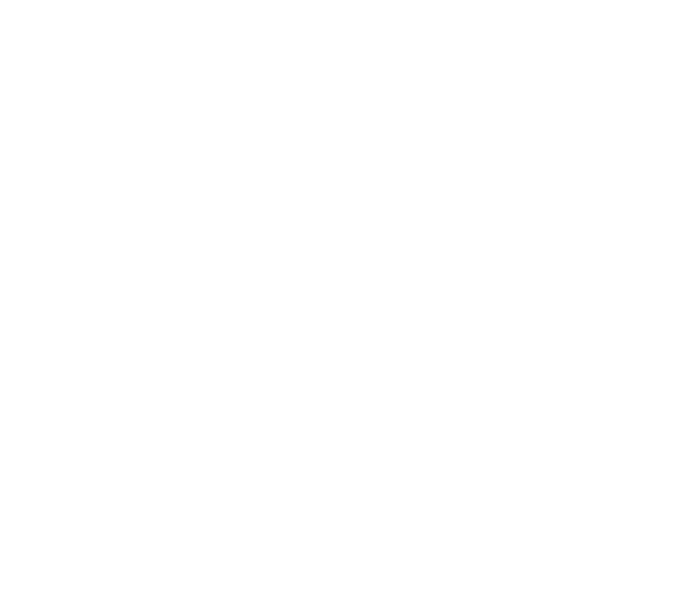2. From the graph
1. Find the amplitude of the wave. (2mks)
2. The period of the wave. (1mk)
3. Find the solution to 3cosx=2 (2mks)
4. A plane leaves an airport A (41.5ºN, 36.4ºW) at 9:00am and flies due north to airport B on latitude 53.2ºN. Taking π as 22/7 and the radius of the earth as 6370Km,
1. Calculate the distance covered by the plane in km (4mks)
2. The plane stopped for 30minutes to refuel at B and flew due east to C, 2500km from B. Calculate:
1. position of C (3mks)
2. The time the plane lands at C if its speed is 500km/h (3mks)
5. The curve given by the equation y=x2+1 is defined by the values in the table below.
1. Complete the table by filling in the missing values. (2mks)
 x 0 0.5 1 1.5 2 2.5 3 3.5 4 4.5 5 5.5 6 y 1 2 5 10 17 26 37
2. Sketch the curve for y=x2+1 for 0≤x≤6 (2mks)
3. Use the mid-ordinate rule with 5 ordinates to estimate the area of the region bounded by the curve y=x2+1, the x-axis, the lines x = 0 and x = 6. (2mks)
4. Use method of integration to find the exact value of the area of the region in (c) above. (2mks)
5. Calculate the percentage error involved in using the mid-ordinate rule to find the area. (2mks)
6.
1. Using a ruler and pair of compasses only construct triangle PQR in which
PQ = 7.5cm QR= 6.0cm and angle PQR = 60º. Measure PR (3mks)
2. On same side of PQ as R
1. Determine the locus of a point T such that angle PTQ = 60º (3mks)
2. Construct the locus of M such that PM = 3.5cm. (2mks)
3. Identify the region W such that PR≥3 and angle PTQ≥60º by shading the unwanted part. (2mks)
7. OABCD is a right pyramid on a rectangular base with AB = 8 cm, BC = 6 cm,
OA = OB = OC = OD = 13 cm. Calculate;
1. the height of the pyramid. (3mks)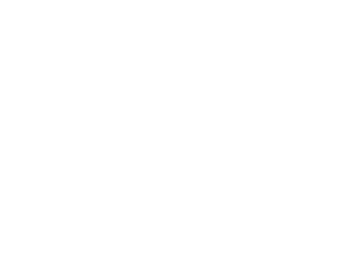2. the inclination of OBC to the horizontal. (2mks)
3. the angle between;
1. OB and DC (3mks)
2. the planes OBC and OAD (2mks)
8. The games master wishes to hire two matatus for a trip. The operators have a Toyota which carries 10 passengers and a Kombi which carries 20 passengers. Altogether 120 people have to travel. The operators have only 20litres of fuel and the Toyota consumes 4 litres on each round trip and the Kombi 1 litre on each round trip. If the Toyota makes x round trips and the kombi y round trips;
1. write down four inequalities in x and y which must be satisfied . (2mks)
2. Represent the inequalities graphically on the grid provided. (3mks)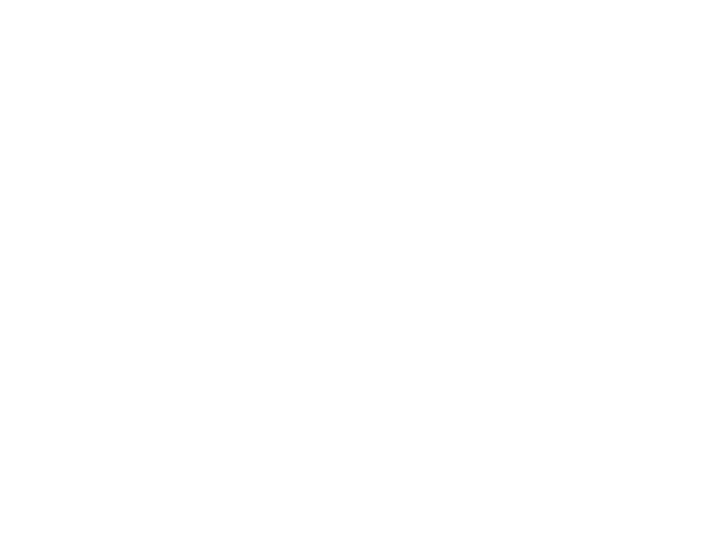3. The operators charge shs.100 for each round trip in the Toyota and shs.300 for each round trip in the kombi;
1. determine the number of trips made by each vehicle so as to make the total cost a minimum. (4mks)
2. find the minimum cost. (1mk)## MARKING SCHEME

SECTION A (50 MARKS)
(Answer all questions in this section in the spaces provided)

1. Use logarithm table to evaluate. (4mks)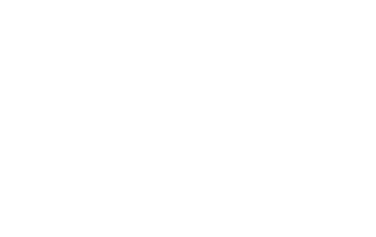2. Three sisters, Ann, Beatrice and Caroline together invested Ksh. 48,000 as capital and started a small business. If the share of profit is Ksh. 2,300, Ksh. 1,700 and Ksh. 800 respectively, shared proportionally. Find the capital invested by each of them. (3mks)
A:B:C
2300:1700:800
23:17:8
23/4817/488/48
Ann = 23/48 x 48000 = sh 23000
Beatrice = 17/48 x 48,000 = sh17000
Caroline = 8/48 x 48000 = sh8000
3. Make t the subject of formula in x=(p+t)1/3 (3mks)
t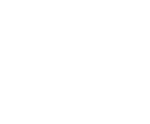x 3 =p + t
1        t
x3t = p + t
x3t - t = p
t(x3 - 1) =   p
(x3 - 1)    x3 - 1
t =   p
(x3 - 1)
4. Without using a calculator or mathematical tables, express      √3      in surd form and simplify. (3mks)
1 - cos30º
(√3) 2
(1 -√3/2)2
2√3
(2+√3)
(2-√3)(2+√3)
4√3 + 6
4 - 3
4√3 + 6
1
=4√3 + 6
5. Expand and simplify (3x-y)4 hence use the first three terms of the expansion to approximate the value of (6-0.2)4. (4mks)
1(3x)(-y)+4(3)(-y)+6(3x)(-y)+4(3x)(-y)+3(3x)(-y)
81x4+-108x3y+54x2y2-12xy3+y4
81x4-108x3y+54x2y2-12xy3+y4
3x=6   -4=-0.2
x=2       y=0.2
81x4-108x3y+54x2y2
81(2)4-(108.8.0.2)+(54.4.0.4)
324-172.8+8.6 4
=159.84
6. Find x without using tables if 3 + log2⁡3 + log2⁡x = log2⁡5 +2 (3mks)
3(log22)+log23+log2x = log25 + 2(log22)
log28 + log23+log2x=log25+log24
log2(8 × 3 × x)= log2(5 x 4)
24x =20 5
24     24
x = 5/6
7. Find the value of m for which the matrix transforms an object into a straight line. (3mks)(m2 x 1)-(2m - 1)1 = 0
m2-(2m-1)= 0
m2-2m+1=0
(m2-m)(m+1)=0
m(m-1)-1(m-1)=0
(m-1)(m-1) = 0
m-1=0     m-1=0
m=1        m=1
8. In the figure below PT is a tangent to the circle at T, PQ = 9cm, SA = 6cm, AT = 8cm and AR = 3cm. Calculate the length of;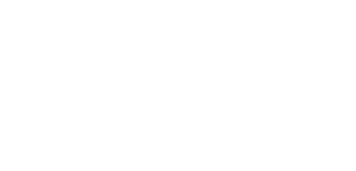1. AQ (2mks)
8 x 6  =3 x Q
3         3
AQ=16cm
2. PT (1mk)
9 x 28 = PT2
PT = √9 x 25
= √252
=15.8745cm
9. A right angled triangle has a base of 15.3 cm and height 7.2 cm, each measured to the nearest 3 mm. Determine the percentage error in finding the area of the triangle, giving your answer to 2 decimal places. (3mks)
Actual area = 15.3 x 2.2 = 110.16
Max area = 15.45 x 2.35 = 113.5575
Min area = 15.15 x 7.25 = 106.8075
(E)= =106.8075 - 113.5575
2
= 6.75 = 3.375
2
%E = (E)  x 100
A.A
=3.375 x 100
0.16
=3.063725490196078
=3.06
10. Given that sin x=0.8, without using a mathematical table and calculator find tan(90-x) (3mks)
sinx= 8/104/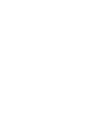tan(92 - x) = 0/A
= 3/4
11. The point B(3,2) maps onto B1(7,1) under a translation T1. Find T1 (2mks)
T1 = T' - T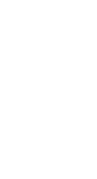12. Using a ruler and a pair of compasses only, construct triangle ABC in which BC=6cm, AB= 8.8cm and angle ABC= 22.5º. (3mks)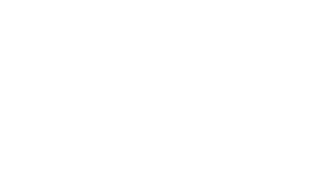13. Two grades of tea A and B, costing sh 100 and 150 per kg respectively are mixed in the ratio 3:5 by mass. The mixture is then sold at sh 160 per kg. Find the percentage profit on the cost price. (3mks)
3/8(100)+5/8(150) = Cost price
37.5 + 93.75 = sh131.25
profit = 100 - 131.25
profit = 100 - 131.25
= sh 28.75
%profit =profit x 100
c.p
= 28.75  x 100
131.25
= 21.904619
= 21.9048%
14. The first, the third and the ninth term of an increasing AP, makes, the first three terms of a G.P. If the first term of the AP is 3, find the difference of the AP and common ratio of GP. (4mks)
a, a+2d, a+8d
3, 3+2d, 3+8d
3+8d =3+2d
3+2d      3
a+24d = a+12d+4d2
0=4d2-12d
0=d(d-3)
4d=0   d-3=0
d=0     d=3
8=3+2(3)
3
=3+6
3
a/3
= 3
15. The matrix M=  maps a triangular object of area 7 square units onto one with area of 35 square units. Find the value of x. (4mks)
def = A.S.F
A.S.F = IA
OA
= 35
7
= 5
5=3y-10
15=3y
y=5
16. The equation of a circle is given by x2+4x+y2-2y-4=0. Determine the centre and radius of the circle (3mks)
x2+4x+(4/2)+y2-2y+(-2/2)2=4+4+1
(x+2)2+(y-1)2=32
(x-a)2+(y-b)2=82
(a,b)=(-2,1) x=3 units

SECTION B (50 MARKS)
(Answer any five questions in this section)

1. A bag contains 3 black balls and 6 white balls. If two balls are drawn from the bag one at a time, find the:
1. Probability of drawing two white balls:
1. With replacement (2mks)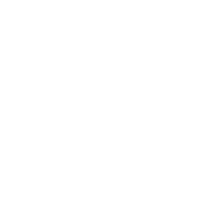2. Without replacement (2mks)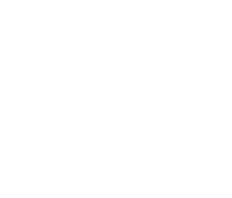2. Probability of drawing a black ball and white ball:
1. With replacement (3mks)
P(BW)   P(WB)
=(1/96/8) + (6/92/8
2/92/94/9
2. Without replacement. (3mks)
1/41/4
= 1/2
2. In the triangle below P and Q are points on OA and OB respectively such that OP:PA = 3 : 2 and OQ : QB = 1 : 2. AQ and PQ intersect at T. Given that OA = a and OB = b.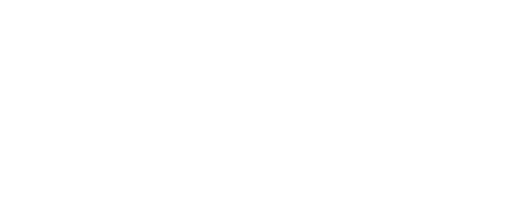1. Express AQ and PQ in terms of a and b. (2mks)
AQ = AQ + OQ
=-a + 1/3b
1/3b - a
PQ = -3/5.a + 1/3b
1/3b - 3/5a
2. Taking BT=kBP and AT=hAQ where h and k are real numbers.
1. Find two expressions for OT in terms of a and b. (2mks)
OT = OA + AT
a+h(1/3b-a)
a+1/3hb - ha
=(1-h)a + 1/3hb
OT = OB + BT
b + k(3/5 a - b)
b + 3/5 ka - kb
(1-k)b + 3/5 ka
2. Use the expression in b(i) above to find the values of h and k. (4mks)
(1 - h) = 3/5k
1/3h = 1-k
h=3-3k
1-(3-3k) = 3/5 k
1-3+3k = 3/5 k
-2 = 3/1k + 3/5 k
k = 5/6
h=3-3k
=3-3(5/6)
= 3 - 5/
h = ½
3. Give the ratio BT:TP. (2mks)
BT:TP
K:1-K
5/6:1-5/6
6 x 5/6 : 1/6 x 6
BT:TP=5:1
3. Complete the table below for the functions y=3cosx-2 for 0º≤x≤360º (2mks)
 x 0 30 60 90 120 150 180 210 240 270 300 330 360 y=3cosx-2 1 -1.5 -4.9 -3.3 0.4 0.1 -3.8 -43.7 -1 1 -2.1 -5 -2.9
1. Plot the graph of y=3cosx-2 in the graph provided below. (3mks)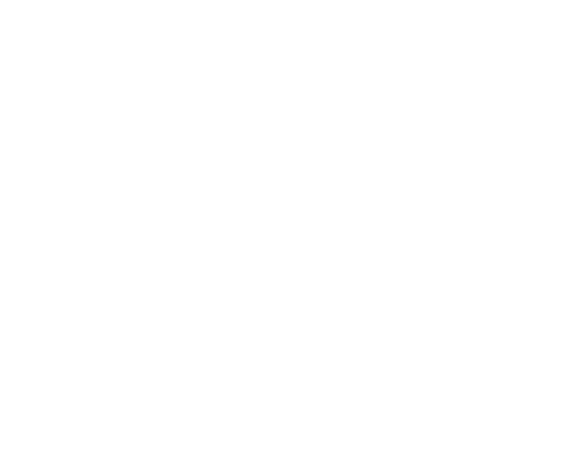2. From the graph
1. Find the amplitude of the wave. (2mks)
1--5 = 3units
2
2. The period of the wave. (1mk)
270º
3. Find the solution to 3cosx=2 (2mks)
3cosx-2=0
18º,117º,150º,249,282
4. A plane leaves an airport A (41.5ºN, 36.4ºW) at 9:00am and flies due north to airport B on latitude 53.2ºN. Taking π as 22/7 and the radius of the earth as 6370Km,
1. Calculate the distance covered by the plane in km (4mks)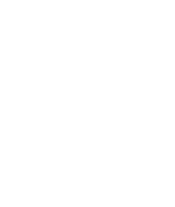Distane =11.7 x 2 x22 x 6370
360          7
=1301.3km
2. The plane stopped for 30minutes to refuel at B and flew due east to C, 2500km from B. Calculate:
1. position of C (3mks)
θ/360 x 2 x 22/7 x 6370cos53.32 = 2500
66.6247θ  2500
66.6247      66.6247
θ = 37.52
36.4 - 22.97 = 13.43º
C(53.2ºN,13.43ºW)
37.52-36.4=1.12º
C(53.2ºN,1.12ºE)
2. The time the plane lands at C if its speed is 500km/h (3mks)
t = d/s
1,301.3
2500
500         500
= (2hrs 36min)+5hrs
=7hrs 36mins
37.52ºx4 = 150.08
2hrs 30mins
5. The curve given by the equation y=x^2+1 is defined by the values in the table below.
1. Complete the table by filling in the missing values. (2mks)
 x 0 0.5 1 1.5 2 2.5 3 3.5 4 4.5 5 5.5 6 y 1 1.25 2 3.25 5 7.25 10 13.25 17 21.25 26 31.25 37
2. Sketch the curve for y=x2+1 for 0≤x≤6 (2mks)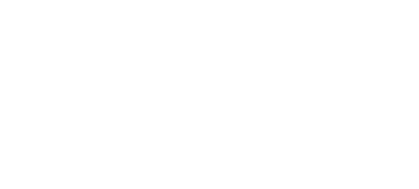3. Use the mid-ordinate rule with 5 ordinates to estimate the area of the region bounded by the curve y=x2+1, the x-axis, the lines x = 0 and x = 6. (2mks)
A=1(1.25+3.25+7.25+13.25+21.25+31.25)
=1(77.5)
=77.5 sqr units
4. Use method of integration to find the exact value of the area of the region in (c) above. (2mks)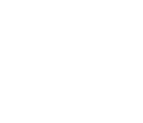(216 + 6 + cc)
3
= 72+6+c-6
=78 sqr units
5. Calculate the percentage error involved in using the mid-ordinate rule to find the area. (2mks)
E = Appr A - Actual A
=77.5 - 78
=0.5sqr units
E =  x 100
A.A
=0.5 x 100
78
=0.64102564125641
=0.6410
6.
1. Using a ruler and pair of compasses only construct triangle PQR in which PQ = 7.5cm QR= 6.0cm and angle PQR = 60º. Measure PR (3mks)
2. On same side of PQ as R
1. Determine the locus of a point T such that angle PTQ = 60º (3mks)
2. Construct the locus of M such that PM = 3.5cm. (2mks)
3. Identify the region W such that PR≥3 and angle PTQ≥60º by shading the unwanted part. (2mks)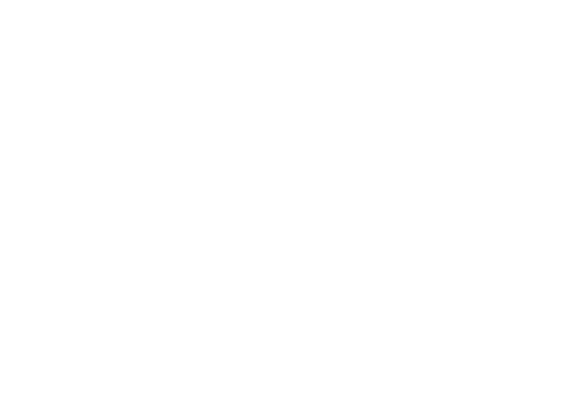7. OABCD is a right pyramid on a rectangular base with AB = 8 cm, BC = 6 cm,
OA = OB = OC = OD = 13 cm. Calculate;
1. the height of the pyramid. (3mks)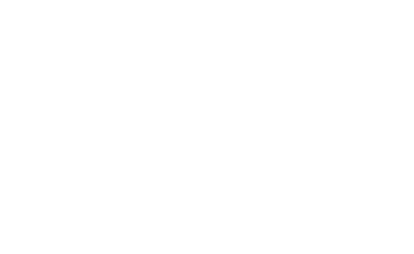2. the inclination of OBC to the horizontal. (2mks)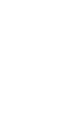Tanθ= 12/4
θ = Tan-13
= 71.57º
3. the angle between;
1. OB and DC (3mks)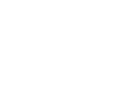cosB = 4/13
B = cos-1 4/13
= 72.08º
2. the planes OBC and OAD (2mks)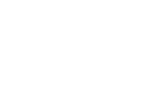Tan = 4/12
Tan-1 4/12
= 18.43º
= 36.87º
8. The games master wishes to hire two matatus for a trip. The operators have a Toyota which carries 10 passengers and a Kombi which carries 20 passengers. Altogether 120 people have to travel. The operators have only 20litres of fuel and the Toyota consumes 4 litres on each round trip and the Kombi 1 litre on each round trip. If the Toyota makes x round trips and the kombi y round trips;
1. write down four inequalities in x and y which must be satisfied . (2mks)
10x + 204 ≥ 120
4x + 4 = 20
x ≥ 0
4 ≥ 0
2. Represent the inequalities graphically on the grid provided. (3mks)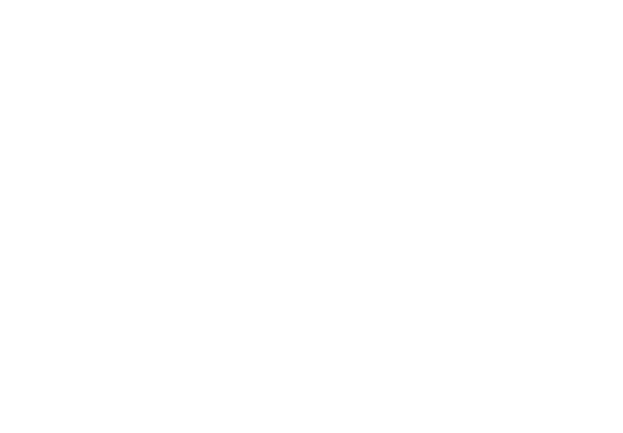3. The operators charge shs.100 for each round trip in the Toyota and shs.300 for each round trip in the kombi;
1. determine the number of trips made by each vehicle so as to make the total cost a minimum. (4mks)
100x + 30y = k
100(1) + 300(10) = k
100 + 3000 = k
k = 5100
100  x + 300y =3100
3100      3100   3100
x   4    = 1
31    10.3
Minimun cost (4,-4)
4 toyota trips
4 kombi trips
2. find the minimum cost. (1mk)
100x + 300y = cosy
100(4) + 300(4) = 400 + 1200
=sh1600

• ✔ To read offline at any time.
• ✔ To Print at your convenience
• ✔ Share Easily with Friends / Students